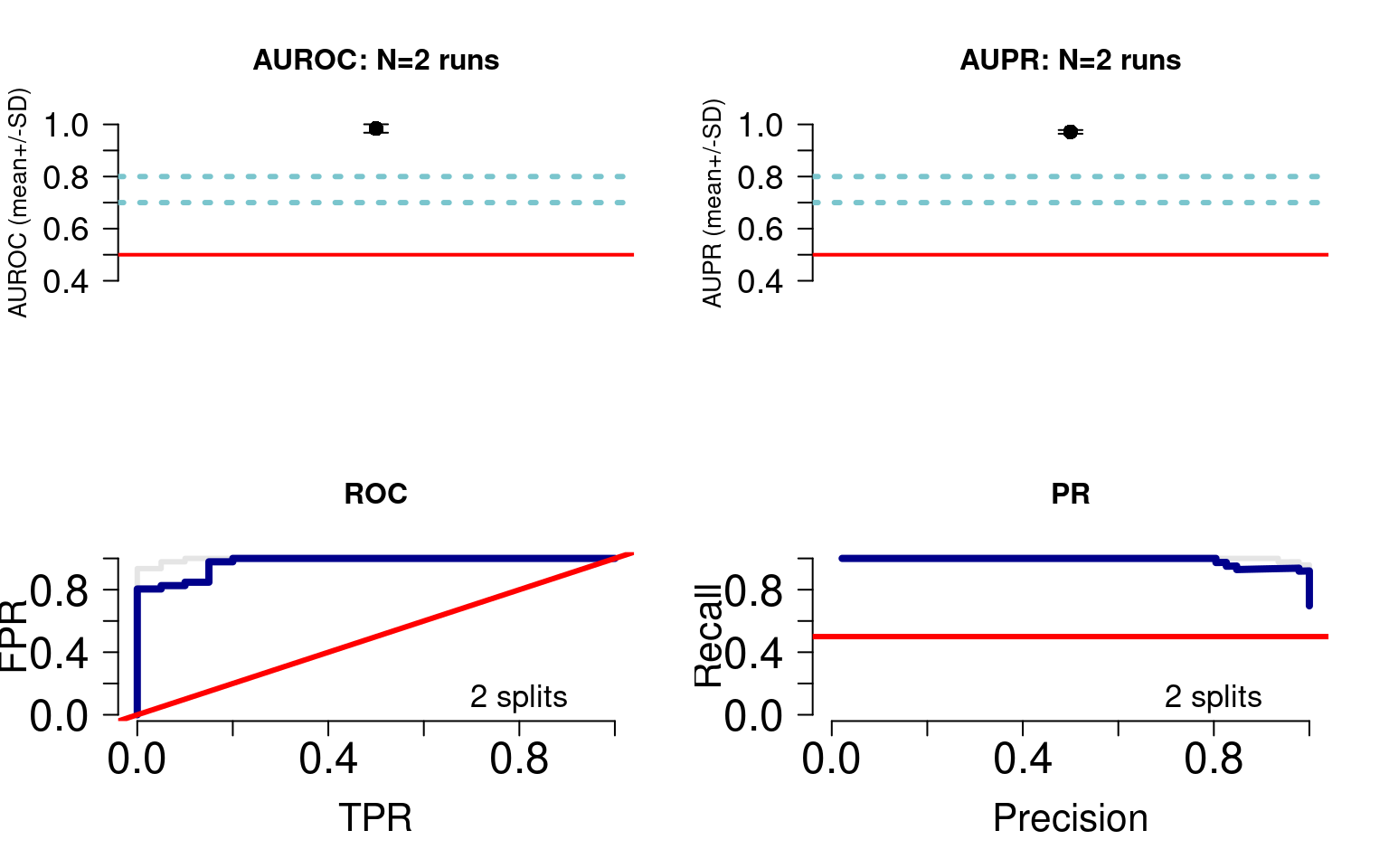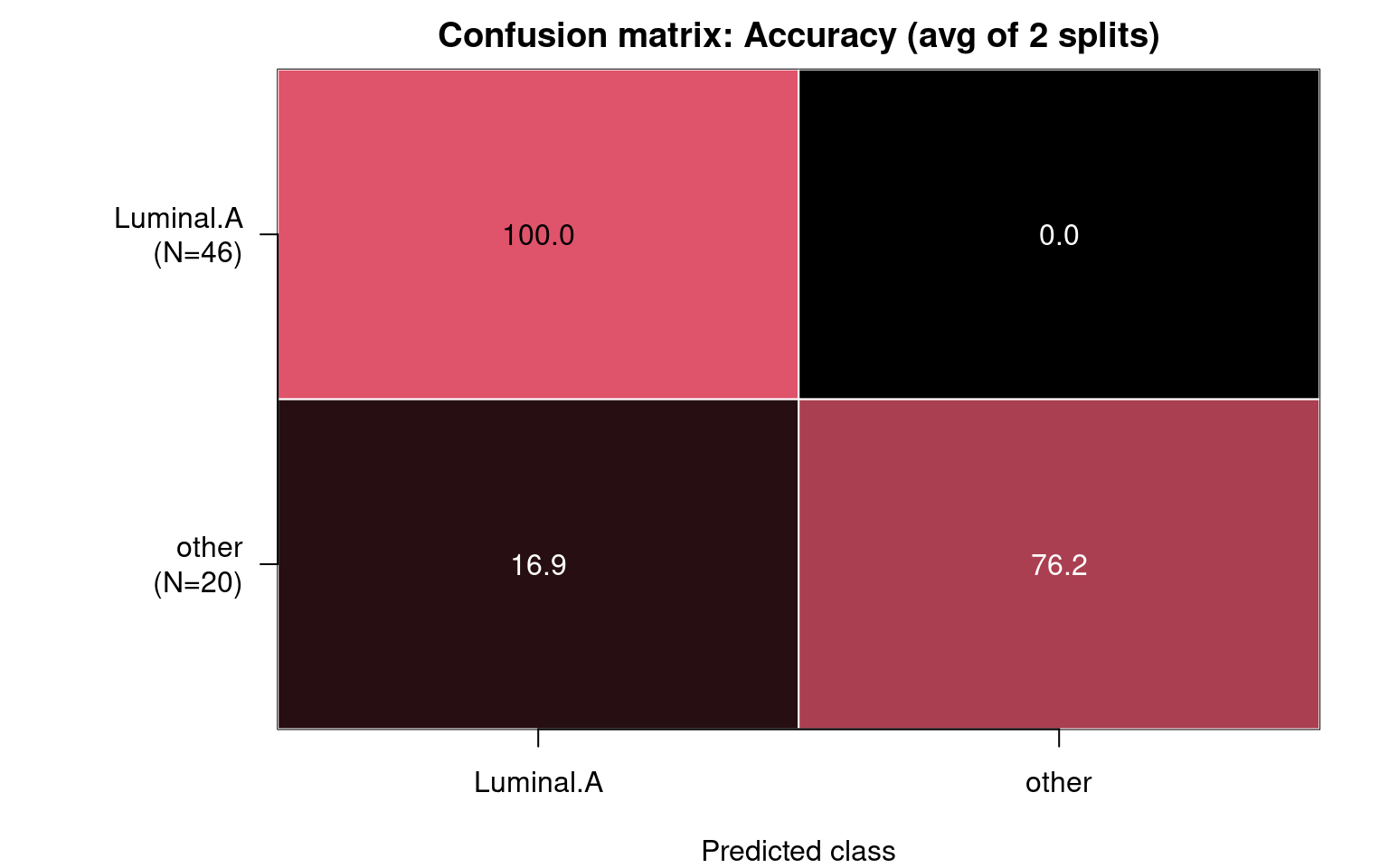# 1 Introduction

In this example we will build a predictor to classify breast tumours as being either of Luminal A subtype or otherwise. The process is identical for classifying three or more labels, and the example uses minimal data for quick runtime.

Although the netDx algorithm requires assay data to be provided in the form of a `MultiAssayExperiment` object, the package comes equipped with the `convertToMAE()` wrapper function to transform raw experimental assay data/tables into a `MultiAssayExperiment` object. We will use data from The Cancer Genome Atlas to build the predictor, converting it from a `MultiAssayExperiment` object into a list to illustrate how to utilize the `convertToMAE()` wrapper function.

We will integrate two types of -omic data:

• gene expression from Agilent mRNA microarrays and
• miRNA sequencing

# 2 Setup

First, we load the `netDx` package.

``suppressWarnings(suppressMessages(require(netDx)))``

# 3 Data

For this example we pull data from the The Cancer Genome Atlas through the BioConductor `curatedTCGAData` package.

``suppressMessages(library(curatedTCGAData))``

We fetch the two layers of data that we need:

``````brca <- suppressMessages(curatedTCGAData("BRCA",
c("mRNAArray",
"miRNASeqGene"),
dry.run=FALSE, version="1.1.38"))``````

The fetch command automatically brings in a `MultiAssayExperiment` object.

``summary(brca)``
``````##               Length                Class                 Mode
##                    2 MultiAssayExperiment                   S4``````

## 3.1 Prepare Data

This next code block prepares the TCGA data. In practice you would do this once, and save the data before running netDx, but we run it here to see an end-to-end example.

``````source("prepare_data.R")
brca <- prepareData(brca,setBinary=TRUE)``````
``````## harmonizing input:
##   removing 28 sampleMap rows with 'colname' not in colnames of experiments``````
``````## harmonizing input:
##   removing 44 sampleMap rows with 'colname' not in colnames of experiments``````

The important thing is to create `ID` and `STATUS` columns in the sample metadata slot. netDx uses these to get the patient identifiers and labels, respectively.

``````pID <- colData(brca)\$patientID
colData(brca)\$ID <- pID``````

# 4 Create feature design rules (patient similarity networks)

To build the predictor using the netDx algorithm, we call the `buildPredictor()` function which takes patient data and variable groupings, and returns a set of patient similarity networks (PSN) as an output. The user can customize what datatypes are used, how they are grouped, and what defines patient similarity for a given datatype. This is done specifically by telling the model how to:

• group different types of data and
• define similarity for each of these (e.g. Pearson correlation, normalized difference, etc.,).

The relevant input parameters are:

• `groupList`: sets of input data that would correspond to individual networks (e.g. genes grouped into pathways)
• `sims`: a list specifying similarity metrics for each data layer

## 4.1`groupList`: Grouping variables to define features

The `groupList` object tells the predictor how to group units when constructing a network. For examples, genes may be grouped into a network representing a pathway. This object is a list; the names match those of `dataList` while each value is itself a list and reflects a potential network.

In this simple example we just create a single PSN for each datatype (mRNA gene expression, and miRNA expression data), containing all measures from that datatype, where measures can be individual genes, proteins, CpG bases (in DNA methylation data), clinical variables, etc.,

``````expr <- assays(brca)
groupList <- list()
for (k in 1:length(expr)) { # loop over all layers
cur <- expr[[k]]; nm <- names(expr)[k]

# all measures from this layer go into our single PSN
groupList[[nm]] <- list(nm=rownames(cur))

# assign same layer name as in input data
names(groupList[[nm]]) <- nm;
}``````

## 4.2`sims`: Define patient similarity for each network

What is this: `sims` is used to define similarity metrics for each layer. This is done by providing a single list - here, `sims` - that specifies the choice of similarity metric to use for each data layer. The `names()` for this list must match those in `groupList`. The corresponding value can either be a character if specifying a built-in similarity function, or a function. The latter is used if the user wishes to specify a custom similarity function.

The current available options for built-in similarity measures are:

• `pearsonCorr`: Pearson correlation (n>5 measures in set)
• `normDiff`: normalized difference (single measure such as age)
• `avgNormDiff`: average normalized difference (small number of measures)
• `sim.pearscale`: Pearson correlation followed by exponential scaling
• `sim.eucscale`: Euclidean distance followed by exponential scaling

In this example, we choose Pearson correlation similarity for all data layers.

``````sims <- list(a="pearsonCorr", b="pearsonCorr")
names(sims) <- names(groupList)``````

# 5 Conversion of raw assay data into MultiAssayExperiment format

Data pulled from The Cancer Genome Atlas through the BioConductor `curatedTCGAData` package automatically fetches data in the form of a `MultiAssayExperiment` object. However, most workflows that might utilize the netDx algorithm will have experimental assay data and patient metadata in the form of data frames/matrices/tables.

To facilitate ease-of-use, the netDx package has a built-in wrapper function `convertToMAE()` that takes in an input list of key-value pairs of experimental assay data and patient metadata, converting it into a `MultiAssayExperiment` object compatible with further analysis using the netDx algorithm. However, all relevant data engineering/preparation should be done before using the `convertToMAE()` wrapper function.

This next code block converts the TCGA data into a list format to illustrate how one might use the `convertToMAE()` wrapper function.

``brcaData <- dataList2List(brca, groupList)``

The keys of the input list of key-value pairs should be labelled according to the type of data corresponding to the value pairs (methylation, mRNA, proteomic, etc) and there must be a key-value pair that corresponds to patient IDs/metadata labelled `pheno`.

``````brcaList <- brcaData\$assays
names(brcaList) <- "pheno"``````

We can now call the `convertToMAE()` wrapper function to convert the list containing experimental assay data and patient metadata into a `MultiAssayExperiment` object.

``brca <- convertToMAE(brcaList)``

We can then proceed with the rest of the netDx workflow.

# 6 Build predictor

Now we’re ready to train our model. netDx uses parallel processing to speed up compute time. Let’s use 75% available cores on the machine for this example. netDx also throws an error if provided an output directory that already has content, so let’s clean that up as well.

``````nco <- round(parallel::detectCores()*0.75) # use 75% available cores
message(sprintf("Using %i of %i cores", nco, parallel::detectCores()))``````
``## Using 54 of 72 cores``
``````outDir <- paste(tempdir(),"pred_output",sep=getFileSep()) # use absolute path
numSplits <- 2L``````

Finally we call the function that runs the netDx predictor. We provide:

• patient data (`dataList`)
• grouping rules (`groupList`)
• list specifying choice of similarity metric to use for each grouping (`sims`)
• number of train/test splits over which to collect feature scores and average performance (`numSplits`),
• maximum score for features in one round of feature selection (`featScoreMax`, set to 10)
• threshold to call feature-selected networks for each train/test split (`featSelCutoff`); only features scoring this value or higher will be used to classify test patients,
• number of cores to use for parallel processing (`numCores`).

The call below runs two train/test splits. Within each split, it:

• splits data into train/test using the default split of 80:20 (`trainProp=0.8`)
• score networks between 0 to 2 (i.e. `featScoreMax=2L`)
• uses networks that score >=9 out of 10 (`featSelCutoff=1L`) to classify test samples for that split.

In practice a good starting point is `featScoreMax=10`, `featSelCutoff=9` and `numSplits=10L`, but these parameters depend on the sample sizes in the dataset and heterogeneity of the samples.

``````t0 <- Sys.time()
set.seed(42) # make results reproducible
model <- suppressMessages(
buildPredictor(
groupList=groupList,    ## grouping strategy
sims = sims,
outDir=outDir,          ## output directory
trainProp=0.8,          ## pct of samples to use to train model in each split
numSplits=2L,           ## number of train/test splits
featSelCutoff=1L,       ## threshold for calling something feature-selected
featScoreMax=2L,        ## max score for feature selection
numCores=nco,           ## set higher for parallelizing
debugMode=FALSE,
keepAllData=FALSE,  ## set to TRUE for debugging or low-level files used by the dictor
logging="none"
))
t1 <- Sys.time()
print(t1-t0)``````
``## Time difference of 10.74017 mins``

# 7 Examine results

Now we get model output, including performance for various train/test splits and consistently high-scoring features.

In the function below, we define top-scoring features as those which score two out of two in at least half of the train/test splits:

``````results <- getResults(model,unique(colData(brca)\$STATUS),
featureSelCutoff=2L,featureSelPct=0.50)``````
``## Detected 2 splits and 2 classes``
``## * Plotting performance``
``## * Compiling feature scores and calling selected features```results` contains `performance`, `selectedFeatures` for each patient label, and the table of feature `scores`.

``summary(results)``
``````##                  Length Class  Mode
## selectedFeatures 2      -none- list
## featureScores    2      -none- list
## performance      4      -none- list``````

Look at the performance:

``results\$performance``
``````## \$meanAccuracy
##  0.9015152
##
## \$splitAccuracy
##  0.9393939 0.8636364
##
## \$splitAUROC
##  0.9956522 0.9728261
##
## \$splitAUPR
##  0.9763790 0.9661563``````

Look at feature scores for all labels, across all train-test splits:

``results\$featureScores``
``````## \$Luminal.A
##                      Feature Split1 Split2
## 1    BRCA_mRNAArray-20160128      2      2
## 2 BRCA_miRNASeqGene-20160128      1      1
##
## \$other
##                      Feature Split1 Split2
## 1    BRCA_mRNAArray-20160128      2      2
## 2 BRCA_miRNASeqGene-20160128      1      2``````

Let’s examine our confusion matrix:

``confMat <- confusionMatrix(model)``Note: Rows of this matrix don’t add up to 100% because the matrix is an average of the confusion matrices from all of the train/test splits.

And here are selected features, which are those scoring 2 out of 2 in at least half of the splits. This threshold is simply for illustration. In practice we would run at least 10 train/test splits (ideally 100+), and look for features that score 7+ out of 10 in >70% splits.

``results\$selectedFeatures``
``````## \$Luminal.A
##  "BRCA_mRNAArray-20160128"
##
## \$other
##  "BRCA_mRNAArray-20160128"    "BRCA_miRNASeqGene-20160128"``````

We finally get the integrated PSN and visualize it using a tSNE plot:

``````## this call doesn't work in Rstudio; for now we've commented this out and saved the PSN file.
psn <- getPSN(brca,groupList,sims = sims,selectedFeatures=results\$selectedFeatures)``````
``## BRCA_miRNASeqGene-20160128: 1 features``
``## BRCA_mRNAArray-20160128: 1 features``
``## * Making integrated PSN``
``## Making nets from sims``
``##  BRCA_miRNASeqGene-20160128``
``## Layer BRCA_miRNASeqGene-20160128: PEARSON CORR``
``## Pearson similarity chosen - enforcing min. 5 patients per net.``
``##  BRCA_mRNAArray-20160128``
``## Layer BRCA_mRNAArray-20160128: PEARSON CORR``
``## Pearson similarity chosen - enforcing min. 5 patients per net.``
``## Net construction complete!``
``````## Warning in dir.create(paste(netDir, "profiles", sep = fsep)): '/tmp/Rtmp52iamk/
``## * Computing aggregate net``
``````##
##  91467 pairs have no edges (counts directed edges)``````
``##  Sparsity = 14809/52975 (28 %)``
``## ``
``## * Prune network``
``## * plotCytoscape is set to FALSE.Set to TRUE to visualize patient network in Cytoscape``
``require(Rtsne)``
``## Loading required package: Rtsne``
``````tsne <- tSNEPlotter(
psn\$patientSimNetwork_unpruned,
colData(brca)
)``````
``## * Making symmetric matrix``
``## * Running tSNE``
``## * Plotting``